" Never place your trust in us，we're only human. "

#  Machine Learning Notes-Linear Regression# Intro

Linear regression predicts a real-valued output based on an input value. In an intuitive way, linear regression is using a straight line to fit arrays of data. Prof.Ng separates this chapter into two parts: One variable and Multiple variables. Basically, cores of two parts are typical, but linear regression with multiple variables introduce matrix into courses. To better understand the course, I think linear algebra is very important.

Examples in this course are my favorite parts. Because of these practical examples, we can as quickly as possible to get into the point. So, I will refer to Professor’s examples in this chapter.

The example is:

If your friend want to rent a house and the rent is impacted by several factors, how do you predict the final rent if your friends tell you his requirement?

Usually, we use x to describe input factors(set area of house as a factor), use y to describe output rent. So the whole procedure on my task is presented below: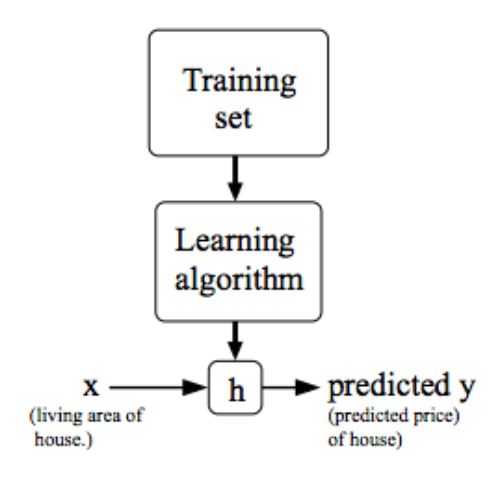If we have only one factor so the variable will be single.

Before talk about definition, a flow chart will be presented below which shows the logic of solving problems.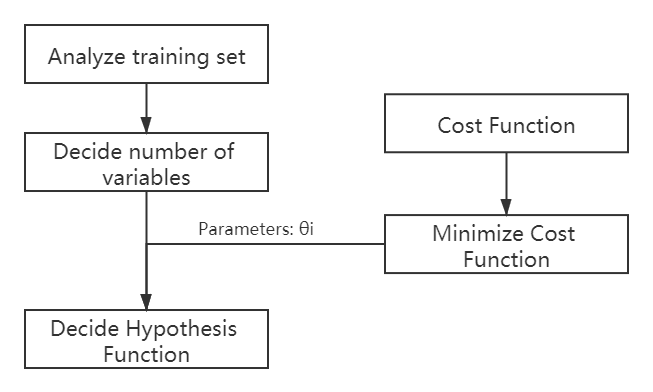## Hypothesis Function

If we want to find a linear line perfectly fit our data sets, we could assume this fit line as hypothesis function. Besides, using polynomial function could also fit data sets well.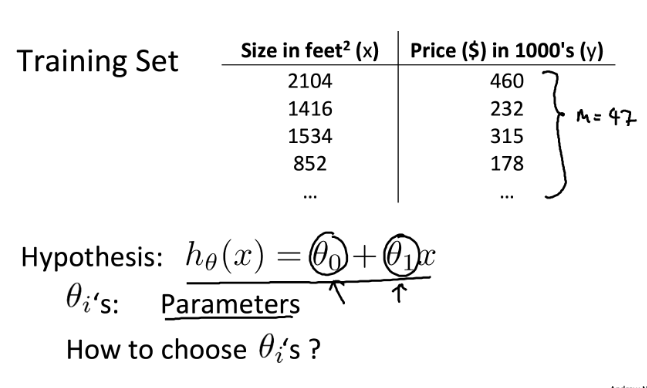## Cost Function

also called “Squared error function”, or “Mean squared error”, or “least square method”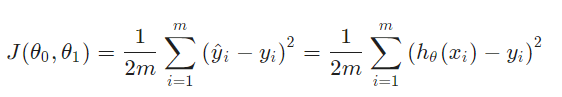• why 1/2 :The mean is halved as a convenience for the computation of the gradient decent (a algorithm which used to calculate the parameter of ML models, the other algorithm is called least square method，in Chinese, it’s called 最小二乘法)
• m: numbers of data set, equals maximum of i.
• Contour Figure: visualize the cost function and find the minimum point. Also used in geography. Because of multiple parameters of cost function(several input data x), using contour figure could be more intuitive.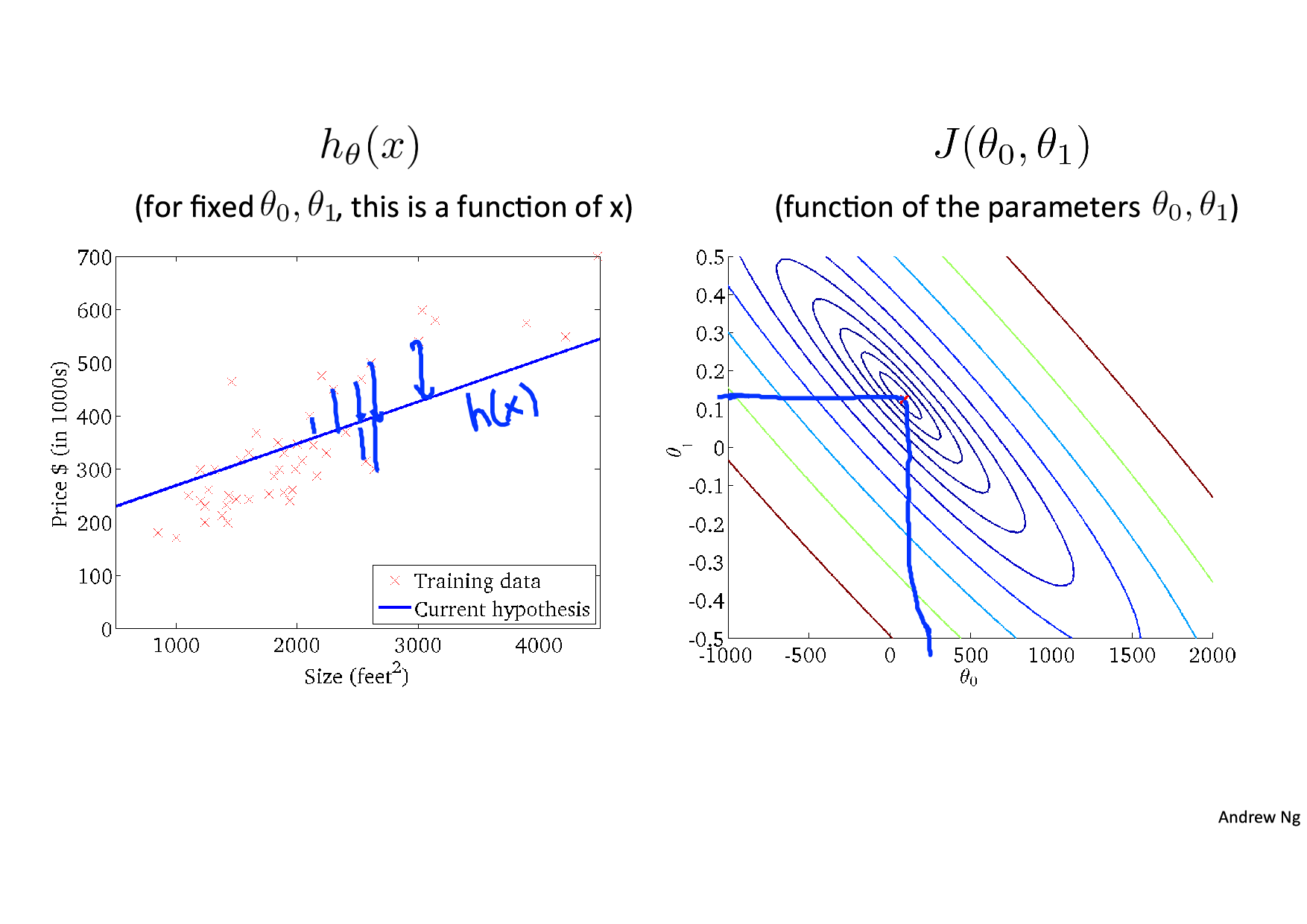## Aim

With hypothesis function and cost function, we could know that our ultimate goal is to Minimize the cost function. Therefore, the line that we assumed could fit data set well. That is why the function is called “cost”, we always want to minimize our cost money.## How to realize it ?

Two ways are mentioned on courses: Gradient Decent and Normal Equation.

Actually, I think some parts of gradient decent is very familiar with Newton-down-hill method. The algorithm is presented below: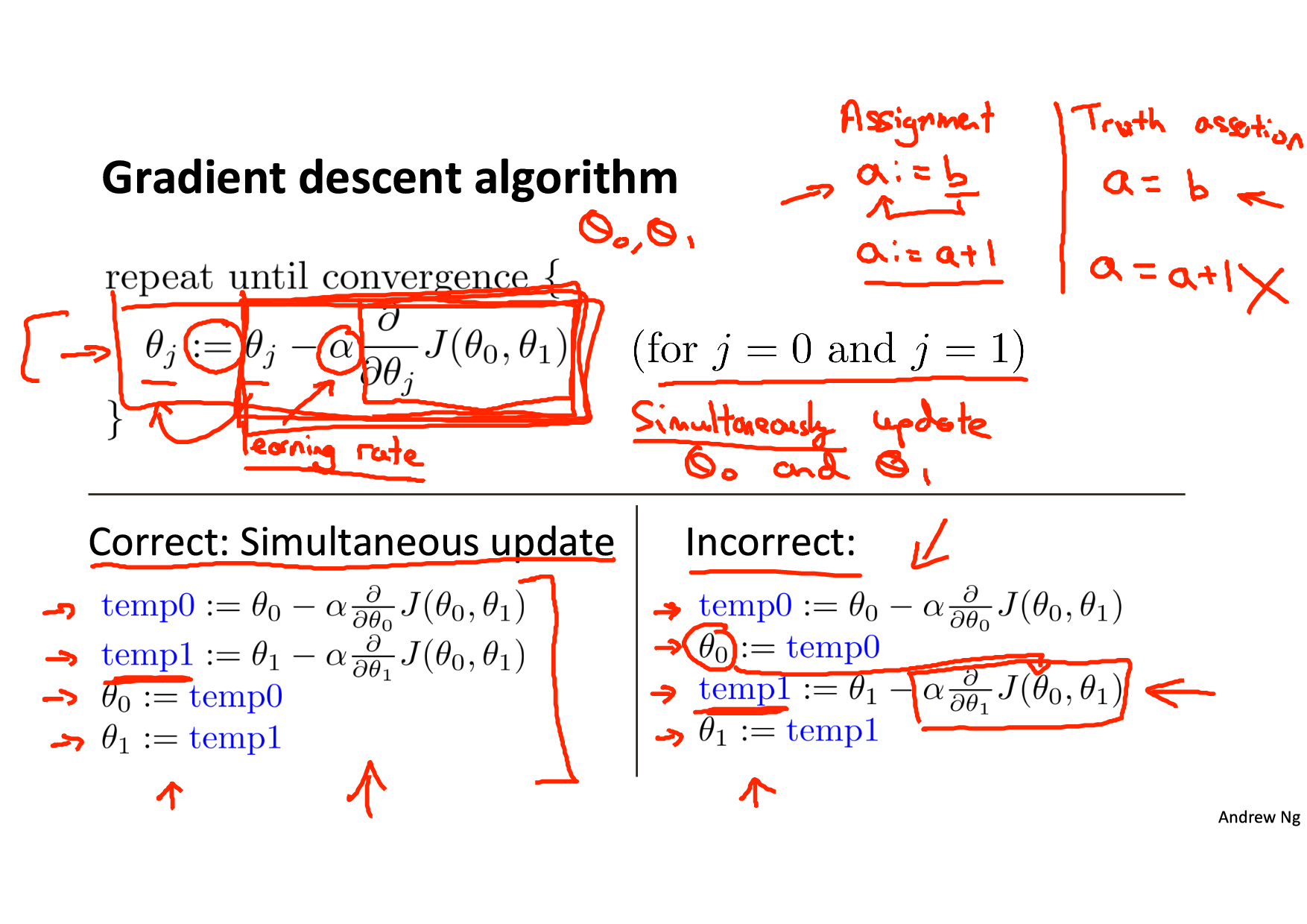If we extend the derivative part of the function we could get function below: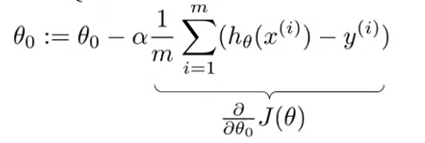The gradient decent means how to find a way to go down the cost function hall and find the minimum point.
:= means a=a+1 in C language.

α means learning rate, if the α is large, the calculating speed would be fast, but it may fail to converge or even diverge. Overshot the minimum.

![Study rate](https://mechhucloud.oss-cn-hangzhou.aliyuncs.com/img/Study rate.png)

Attention

• the θ~0~ and θ~1~ should be updated simultaneously .
• derivative will be smaller as we approach a local minimum, so no need to decrease α over time.
• the cost function is convex function.
• batch: each step of gradient decent uses all the training examples.

### Normal Equation

The normal equation formula is presented below:

![Normal Equation Formula](https://mechhucloud.oss-cn-hangzhou.aliyuncs.com/img/Normal Equation Formula.png)

In this formula, X represent factors, θ is already the minimum θ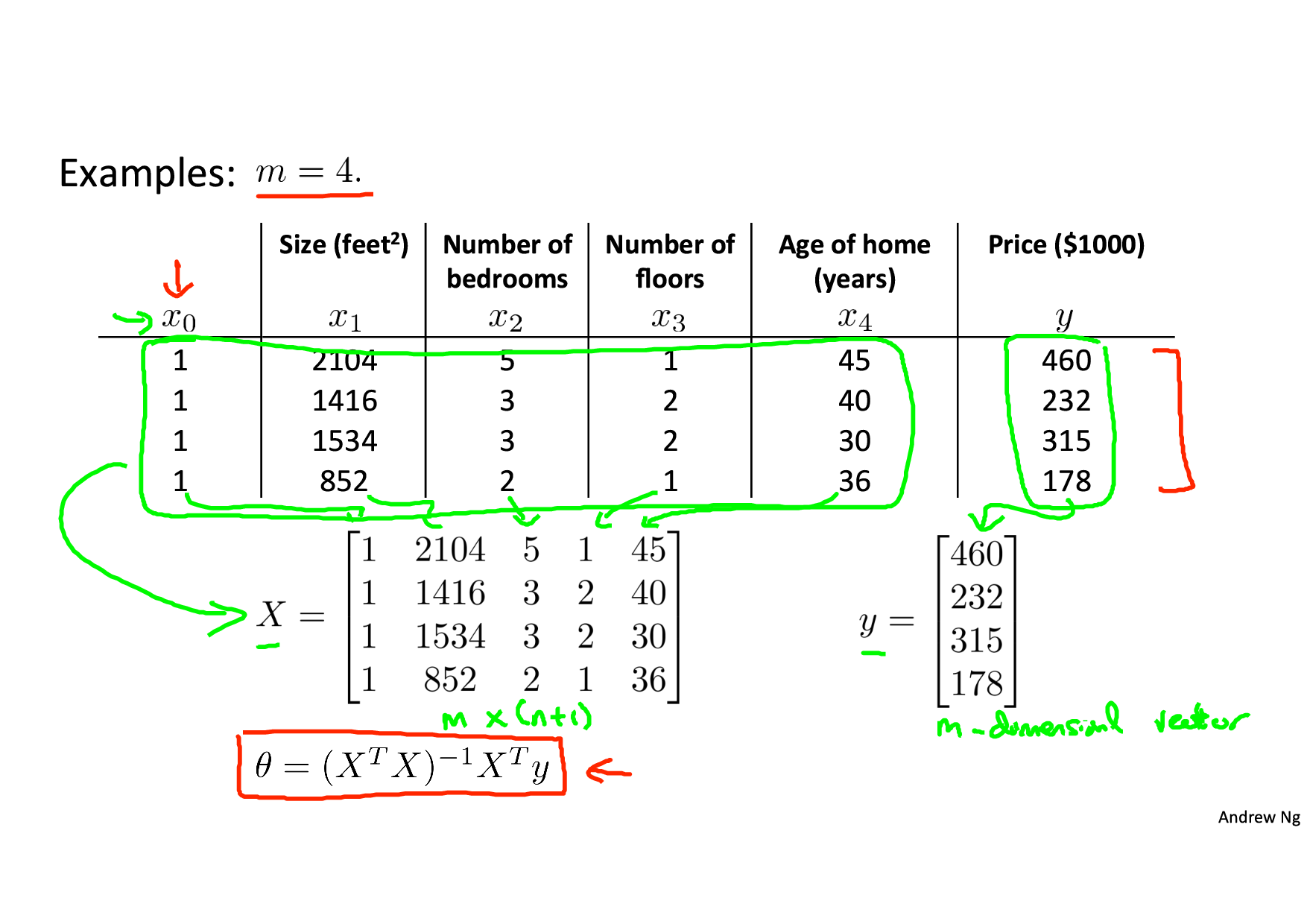## Contrast:

the pros and cons of gradient decent and normal equation

Need to choose alpha No need to choose alpha
Needs many iterations No need to iterate
O (kn^2*k**n*2) O (n^3n3), need to calculate inverse of X^TXXT**X*
Works well when n is large Slow if n is very large(n<10000,recommend)

• 1134
• 1

### YOU MIGHT ALSO LIKE

0 0 vote
Article Rating
Subscribe1 评论
Inline Feedbacks